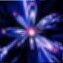# How To Pass Programs From My Classpad To Mi Pc

### #1girdeux

girdeux

•• Members
•• 88 posts
• Location:Spain / Castell?n

• Calculators:
casio fx-115ms;

Posted 04 August 2006 - 02:53 PM

The problem is that with classpad manager I can pass all my programs and variables to my PC, but in only one file .mcs (all programs and variables in this file). I want to pass only one program to my PC. Someone can help me. Thank you.

I am studing chemical engineering, and I have this programs:

Transport phenomena

temp_o: It calculates adimensional temperature for an infinite laminae.

To introduce: Biot, Fouriere, adimensional position; only for infinite laminae. Less time takes but or depending
on the given values.

cof_trns: It calculates coefficients of transport; like Nusselt and Sherwood.

To introduce Reynolds, Prand and Smith. It calculates the module of Nusselt and Serwood, in a certain point or avarage.

Basic operations of flow of fluids

Moody: It calculates the factor of Fanning and Darcy.

To introduce relative rugosity and the module of Reynolds. It calculates the factor of Fanning (f) and the factor
of Darcy (4?f) by means of the equation of Chen.

Y1: It calculates the factor of correction of gases.

Factor of correction that considers the variation of the density of gases with the pressure.
To introduce K=Cp/Cv, B=D2/D1, P2/P1.

Mathematical analysis

AproxPic: It constructs the subsequent approaches of Picard of the solution of a problem of Cauchy.

To introduce the function, initial x, initial y, and the number of approaches of Picard.

erPicard: It considers the error that is committed in the approaches of Picard.

To introduce: K, r, M, and the maximum number of approaches of Picard.

poliEuler: It constructs polygonal of Euler associated to a problem of Cauchy.

To introduce: r, M, initial x, and -1 or 1 respectively if it is wanted that the polynomial is constructed towards
the left or the right of the starting point.

### #2MicroPro

MicroPro

Casio Overlord

•• Deputy
•• 640 posts
• Gender:Male
• Location:Iran

• Calculators: